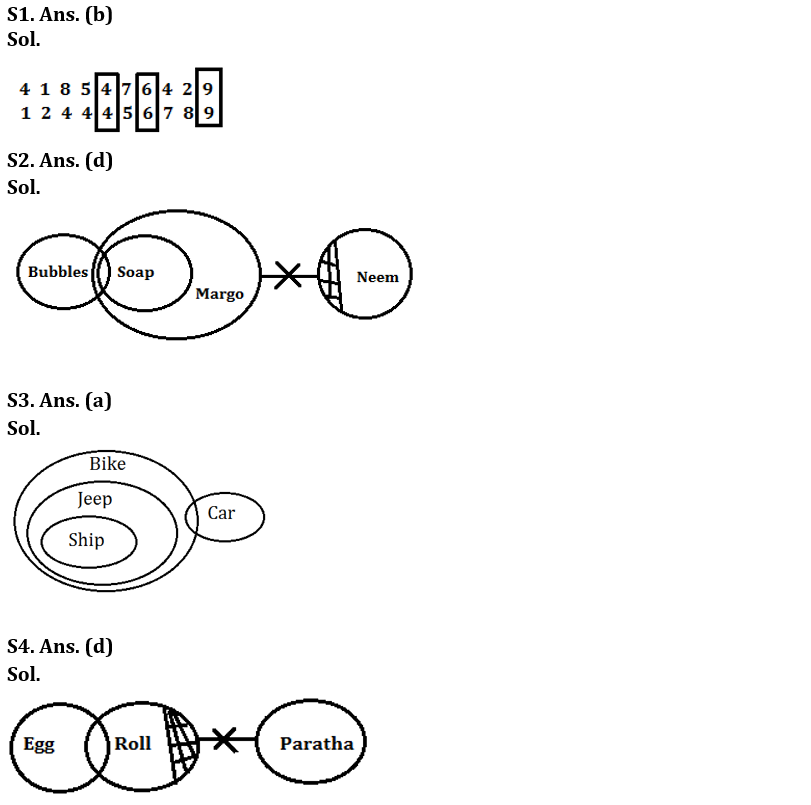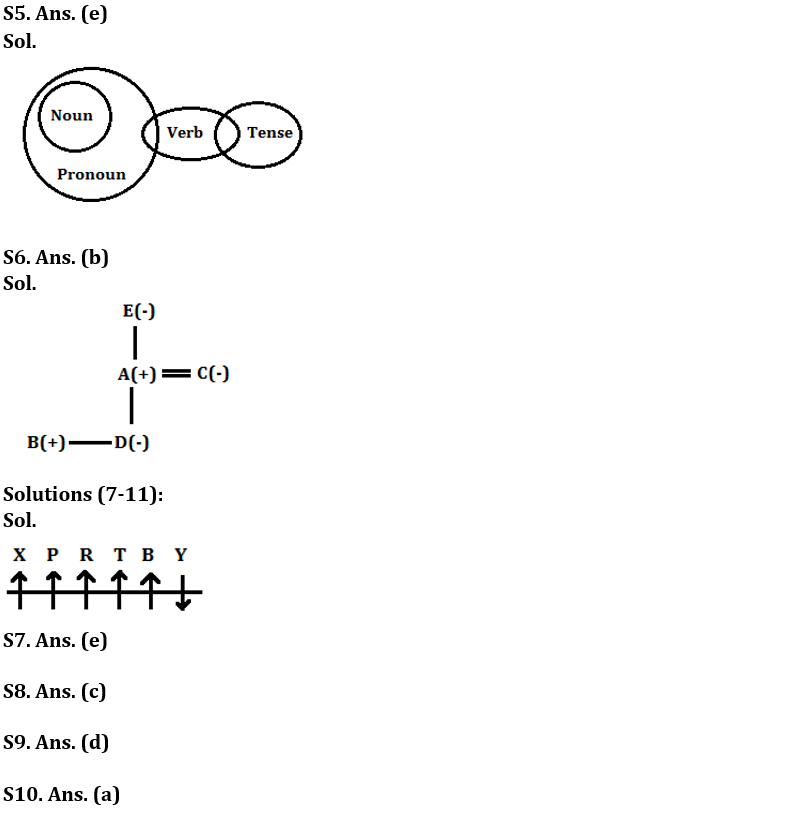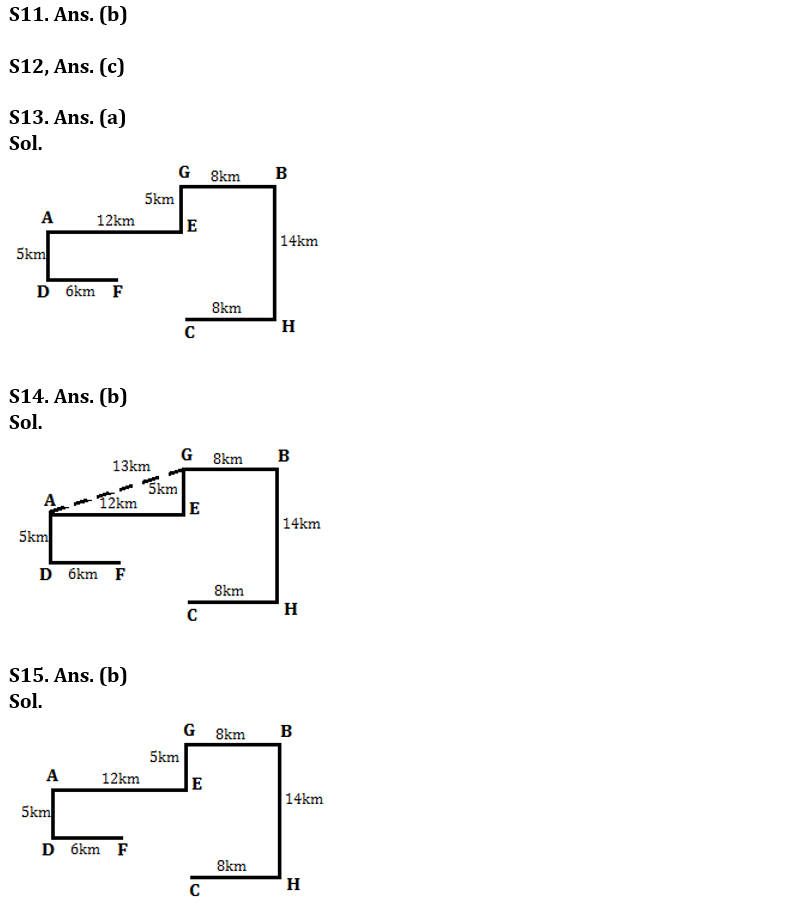Latest Banking jobs   »   Reasoning Ability Quiz For Bank Foundation...

# Reasoning Ability Quiz For Bank Foundation 1st January

Q1. How many such numerals are there in the number ‘4185476429’ which will remain at the same position when arranged in ascending order from left to right?
(a) None
(b) Three
(c) More than three
(d) Two
(e) None of these

Direction (2-5): In each of the questions below some statements are given followed by some conclusions. You have to take the given statements to be true even if they seem to be at variance with commonly known facts. Read all the conclusions and then decide which of the given conclusions logically follows from the given statements disregarding commonly known facts.

Q2. Statements: Only a few Bubbles are Soap.
All Soap are Margo.
Some Neem are not Margo.
Conclusions: I. Some Bubbles are not Neem.
II. Some Soap are not Bubbles.

(a) If only conclusion I follows.
(b) If only conclusion II follows.
(c) If either conclusion I or II follows.
(d) If neither conclusion I nor II follows.
(e) If both conclusions I and II follow.

Q3. Statements: All Ship are Jeep.
All Jeep are Bike.
Some Car are Bike.
Conclusions: I. Some Ship can be Car.
II. All Car are Bike.

(a) If only conclusion I follows.
(b) If only conclusion II follows.
(c) If either conclusion I or II follows.
(d) If neither conclusion I nor II follows.
(e) If both conclusions I and II follow.

Q4. Statements: Some Egg are Roll.
Some Roll are not Paratha.
Conclusions: I. All Egg can never be Paratha.
II. Some Paratha are not Roll.

(a) If only conclusion I follows.
(b) If only conclusion II follows.
(c) If either conclusion I or II follows.
(d) If neither conclusion I nor II follows.
(e) If both conclusions I and II follow.

Q5. Statements: All Noun are Pronoun.
Some Pronoun are Verb.
Some Verb are Tense.
Conclusions: I. Some Noun can be Verb.
II. Some Tense being Pronoun is a possibility.

(a) If only conclusion I follows.
(b) If only conclusion II follows.
(c) If either conclusion I or II follows.
(d) If neither conclusion I nor II follows.
(e) If both conclusions I and II follow.

Q6. In the five family members – A is the Father of B’s sister. E is the paternal grandmother of D. B is the only son of C. Find the relation of D with respect to A?
(a) Sister
(b) Daughter
(c) Daughter in law
(d) Mother
(e) None of these

Direction (7-11): Study the following information carefully and answer the questions given below:
Six persons are sitting in a row Some of them are facing to the north while rest are facing to South. P sits 3rd to the left of B and both does not sit at the extreme ends. The persons who sit at the extreme ends are facing opposite direction. There are two persons sit between X and T. X does not sit near to B. R sits 3rd to the right of Y who is an immediate neighbor of B. R faces to the north. Y faces opposite direction with respect to R. Both P and T are facing in the same direction as R.

Q7. How many persons are facing to the north?
(a) Two
(b) Three
(c) One
(d) Four
(e) Five

Q8. Four of the following five are alike in a certain way and hence they form a group. Which one of the following does not belong to that group?
(a) P
(b) T
(c) Y
(d) B
(e) R

Q9. What is the position of X with respect to R?
(a) 3rd to the left
(b) 2nd to the right
(c) immediate to the left
(d) 2nd to the left
(e) None of these

Q10. How many persons are sit right side from T?
(a) Two
(b) One
(c) Three
(d) Four
(e) None of these

Q11. Who among the following persons are sitting at extreme end?
(a) P-X
(b) X-Y
(c) P-T
(d) B-X
(e) R-Y

Direction (12-15): Study the following information carefully and answer the questions given below:
Point G is in 5km north of Point E. Point B is in 8km east of Point G. Point C is in 8km west of Point H. Point B is in 14km north of Point H. Point D is in 6km west of Point F. Point A is in 5km north of Point D. Point E is in 12km east of Point A.

Q12. What is the total distance between Point A and Point B?
(a) 22km
(b) 2500m
(c) 25km
(d) 20km
(e) None of these

Q13. What is the direction of Point D with respect to Point G?
(a) South-west
(b) South-east
(c) North
(d) South
(e) North-west

Q14. What is the shortest distance between Point A and Point G?
(a) 11km
(b) 13km
(c) 14km
(d) 18km
(e) None of these

Q15. What is the direction of Point D with respect to Point C?
(a) South-east
(b) North-west
(c) South-west
(d) South
(e) North

Solutions#### Congratulations!